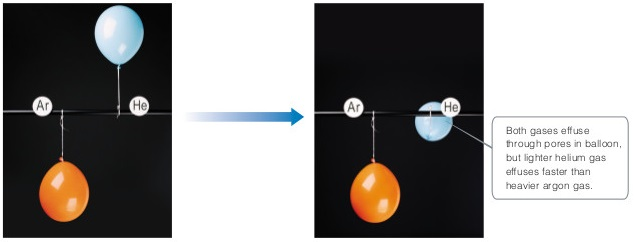# Problem: An illustration of Grahams law of effusion.Because pressure and temperature are constant in this figure but volume changes, which other quantity in the ideal-gas equation must also change?

###### FREE Expert Solution

ideal gas law: PV = nRT

constant P & T & R

85% (416 ratings)###### Problem DetailsAn illustration of Grahams law of effusion.

Because pressure and temperature are constant in this figure but volume changes, which other quantity in the ideal-gas equation must also change?

Frequently Asked Questions

What scientific concept do you need to know in order to solve this problem?

Our tutors have indicated that to solve this problem you will need to apply the Effusion concept. You can view video lessons to learn Effusion. Or if you need more Effusion practice, you can also practice Effusion practice problems.